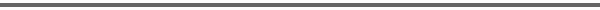Journal Home Page Cumulative Index List of all Volumes Complete Contentsof this Volume Previous Article Next Article Journal for Geometry and Graphics 7 (2003), No. 1, 041--052 Copyright Heldermann Verlag 2003A Voronoi Poset Roderik C. Lindenbergh Mathematical Geodesy and Positioning, Delft University of Technology, Kluyverweg 1, 2629 HS Delft, The Netherlands, r.c.lindenbergh@geo.tudelft.nlGiven a set S of n points in general position, we consider all k-th order Voronoi diagrams on S, for k = 1,...,n, simultaneously. We recall symmetry relations for the number of cells, number of vertices and number of circles of certain orders. We introduce a poset Π(S) that consists of the k-th order Voronoi cells for all k = 1,...,n, that occur for some set S. We prove that there exists a rank function on Π(S) and moreover that the number of elements of odd rank equals the number of elements of even rank of Π(S), provided that n is odd. Keywords: k-th order Voronoi diagrams, k-sets, posets, point configurations. MSC: 52B55, 68U05. FullText-pdf (152 KB) for subscribers only.